﻿ 一种基于同心双曲线相交的多波束测深归位算法
«上一篇文章快速检索 高级检索

 哈尔滨工程大学学报2020, Vol. 41Issue (6): 922-928  DOI: 10.11990/jheu.2019030200

### 引用本文WU Dongqiang, BU Xianhai, XU Fangzheng, et al. Multi-beam footprint reduction algorithm in an echo sounder system based on the intersection of co-concentric hyperbolas[J]. Journal of Harbin Engineering University, 2020, 41(6): 922-928. DOI: 10.11990/jheu.201903020.### 文章历史

1. 山东科技大学 测绘科学与工程学院, 山东 青岛 266590;
2. 自然资源部海岛(礁)测绘技术重点实验室, 山东 青岛 266590

Multi-beam footprint reduction algorithm in an echo sounder system based on the intersection of co-concentric hyperbolas
WU Dongqiang 1, BU Xianhai 1, XU Fangzheng 1, FENG Chengkai 1, YANG Fanlin 1,2
1. College of Geodesy and Geomatics, Shandong University of Science and Technology, Qingdao 266590, China;
2. Key Laboratory of Surveying and Mapping Technology on Island and Reef, Ministry of Natural Resources, Qingdao 266590, China
Abstract: Existing multi-beam footprint reduction algorithms mostly ignore the changes in attitude and heading during the round trip of a beam, and some models are relatively complex, which bring errors into the final results. To solve these problems, in this paper, a new algorithm based on the intersection of co-concentric hyperbolas, in which the transmitted beam energy and received beam energy are abstracted as two regular cones that share the same vertex, is proposed. Thus, the footprints of wave beams are products of the intersecting hyperbolas, which are formed by the projection of two cones on the seabed. Finally, by solving the equations of the intersecting hyperbolas, the coordinates of the footprints are acquired. To verify the effectiveness of the proposed method, data from shallow water and mid-deep water are processed by the conventional algorithm, virtual concentric array algorithm, and proposed algorithm. Moreover, by comparing the results and depth discrepancy of overlapping regions, the proposed algorithm was found to be consistent with the results of the virtual concentric array algorithm, and they have the same level of accuracy. The proposed algorithm is also more accurate than the conventional algorithm and has a significant effect on improving the accuracy of multi-beam data processing.
Keywords: multi-beam echo sounder system    footprint reduction    footprints    transducer orientation    cones    co-concentric    hyperbolas    virtual intersection

1 同心双曲线相交模型构建 1.1 相关坐标系定义

1.2 双曲线相交归位原理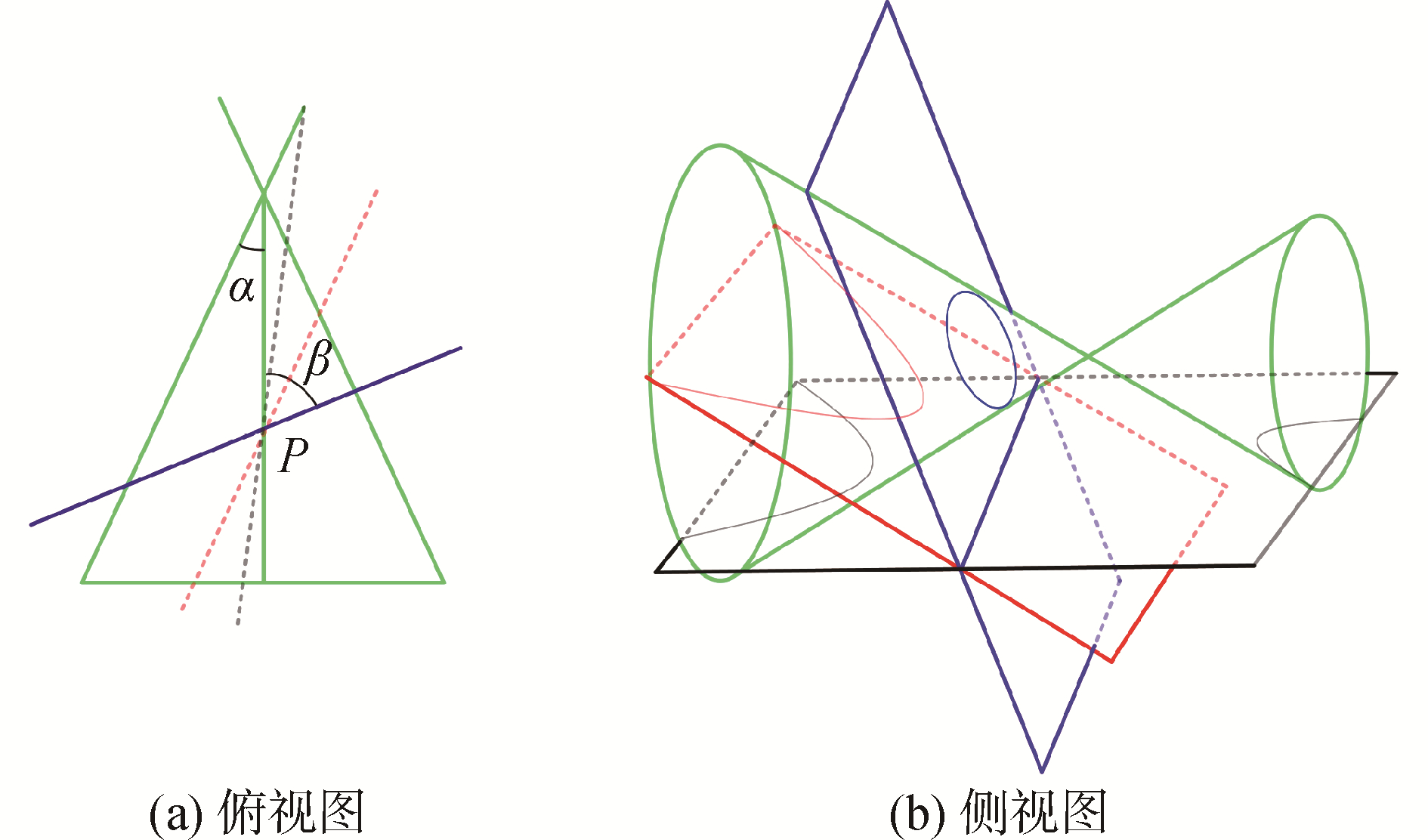Download: 图 1 平面与圆锥面相交得到不同的圆曲线 Fig. 1 Different curves formed with different planes intersecting the cone
2 同心双曲线相交模型的波束向量计算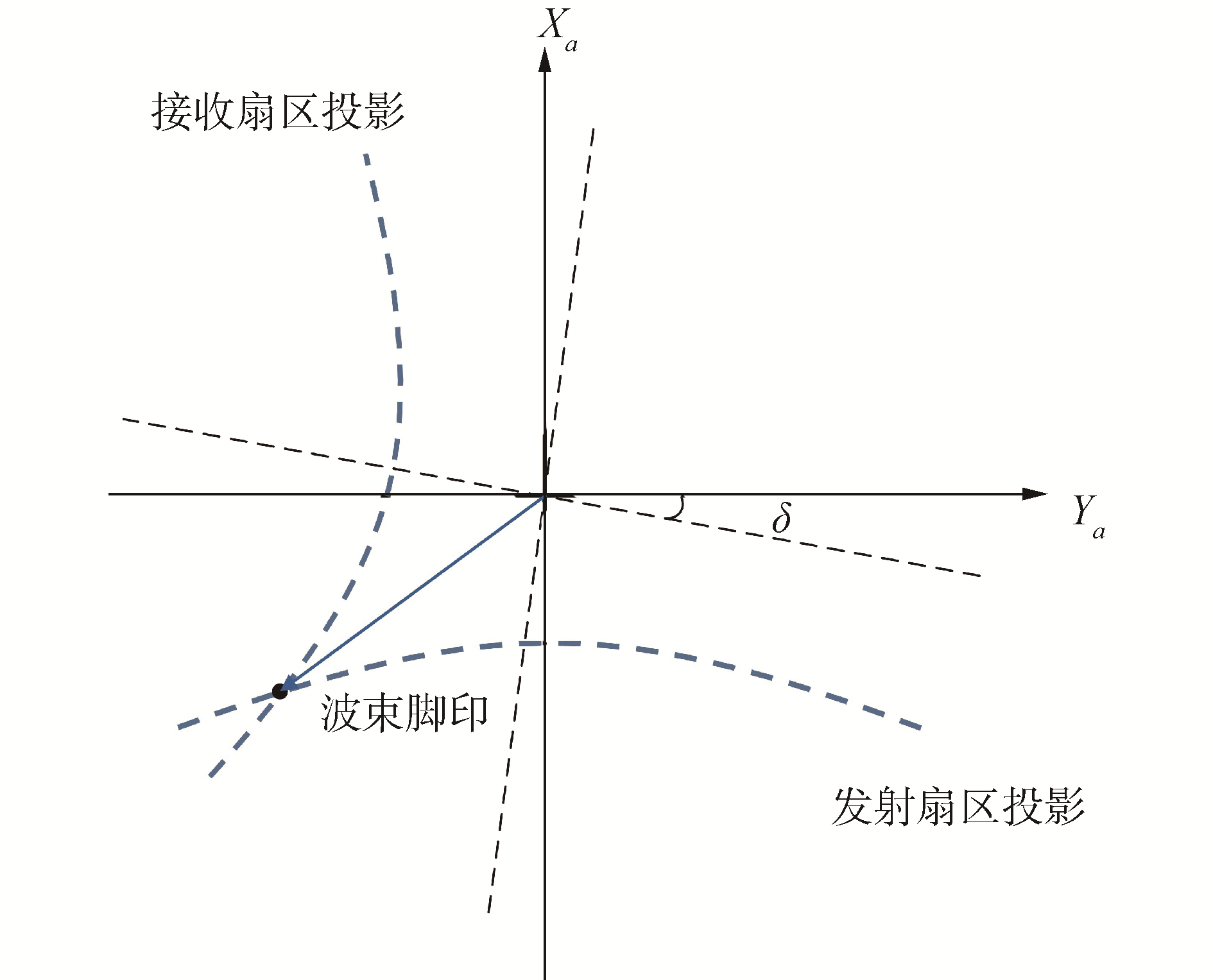Download: 图 2 本文算法中波束脚印表示方式 Fig. 2 Description of footprints formed by the intersection of two hyperbolas in this paper

 ${\left\{ {\begin{array}{*{20}{l}} {{x_t} = {a_t}\cosh {t_1}}\\ {{y_t} = {b_t}\sinh {t_1}} \end{array}} \right.}$ (1)
 ${\left\{ {\begin{array}{*{20}{l}} {{x_r} = {a_r}\cosh {t_2}}\\ {{y_r} = {b_r}\sinh {t_2}} \end{array}} \right.}$ (2)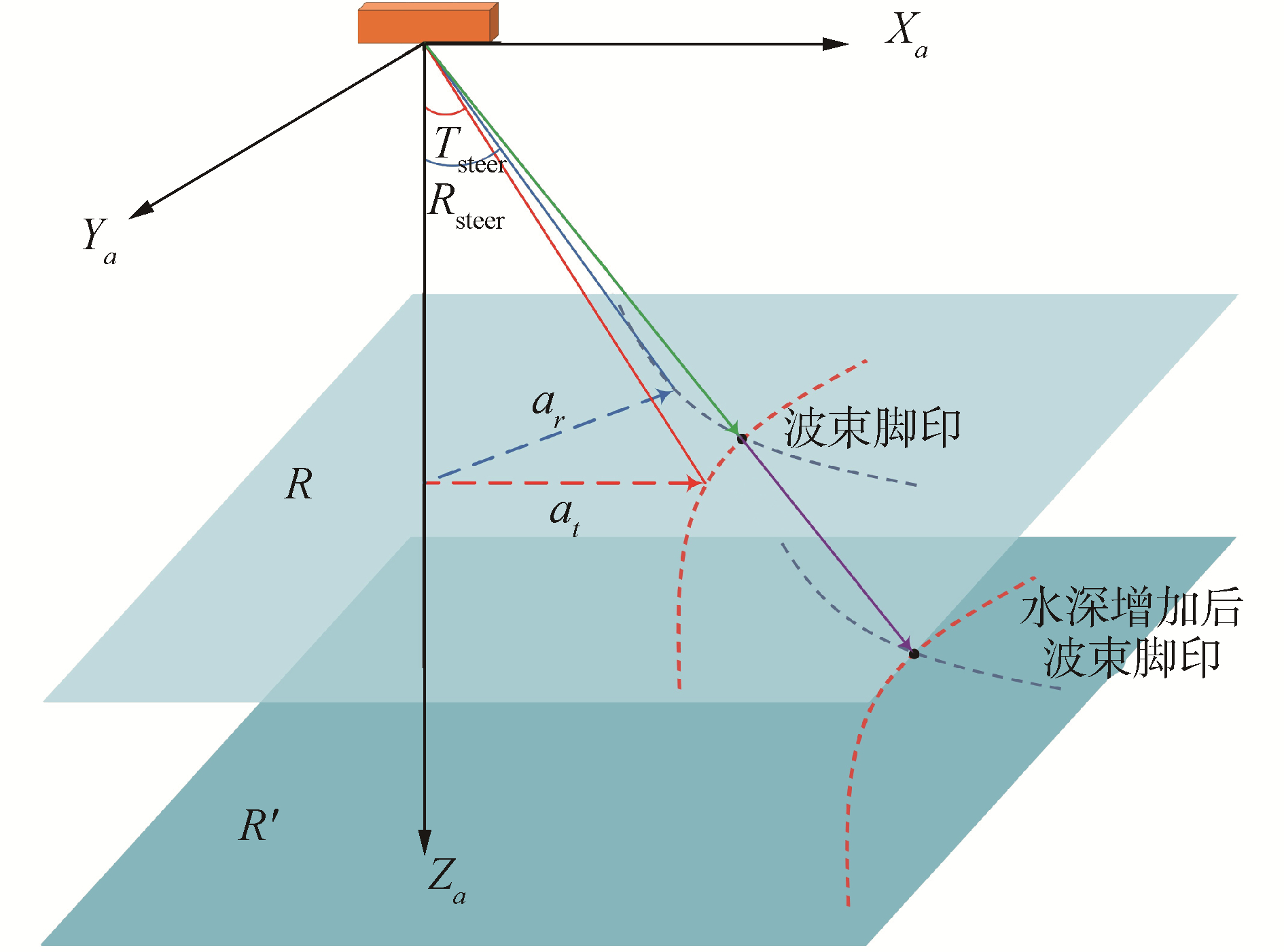Download: 图 3 双曲线参数定义 Fig. 3 Parameter definition of the hyperbola

 $\left\{\begin{array}{l} a_{t}=\tan T_{\text {steer }} \\ b_{t}=1 \\ a_{r}=\tan R_{\text {steer }} \\ b_{r}=1 \end{array}\right.$ (3)

 $\delta=\arccos \left(\boldsymbol{T}_{x} \cdot \boldsymbol{R}_{x}\right)-\frac{\pi}{2}$ (4)

 $\left\{\begin{array}{l} x_{t}=a_{t} \cosh t_{1} \\ y_{t}=b_{t} \sinh t_{1} \\ x_{r}=b_{r} \cos \delta \sinh t_{2}-a_{r} \sin \delta \cosh t_{2} \\ y_{r}=b_{r} \sin \delta \sinh t_{2}+a_{r} \cos \delta \cosh t_{2} \end{array}\right.$ (5)

 $\left\{\begin{array}{l} \theta_{1}=\arctan \left(\sqrt{\left(x_{L}^{2}+y_{L}^{2}\right)} / z_{L}\right) \\ \theta_{2}=\arctan \left(x_{L} / y_{L}\right) \end{array}\right.$ (6)

3 实验与分析

3.1 浅水数据实验分析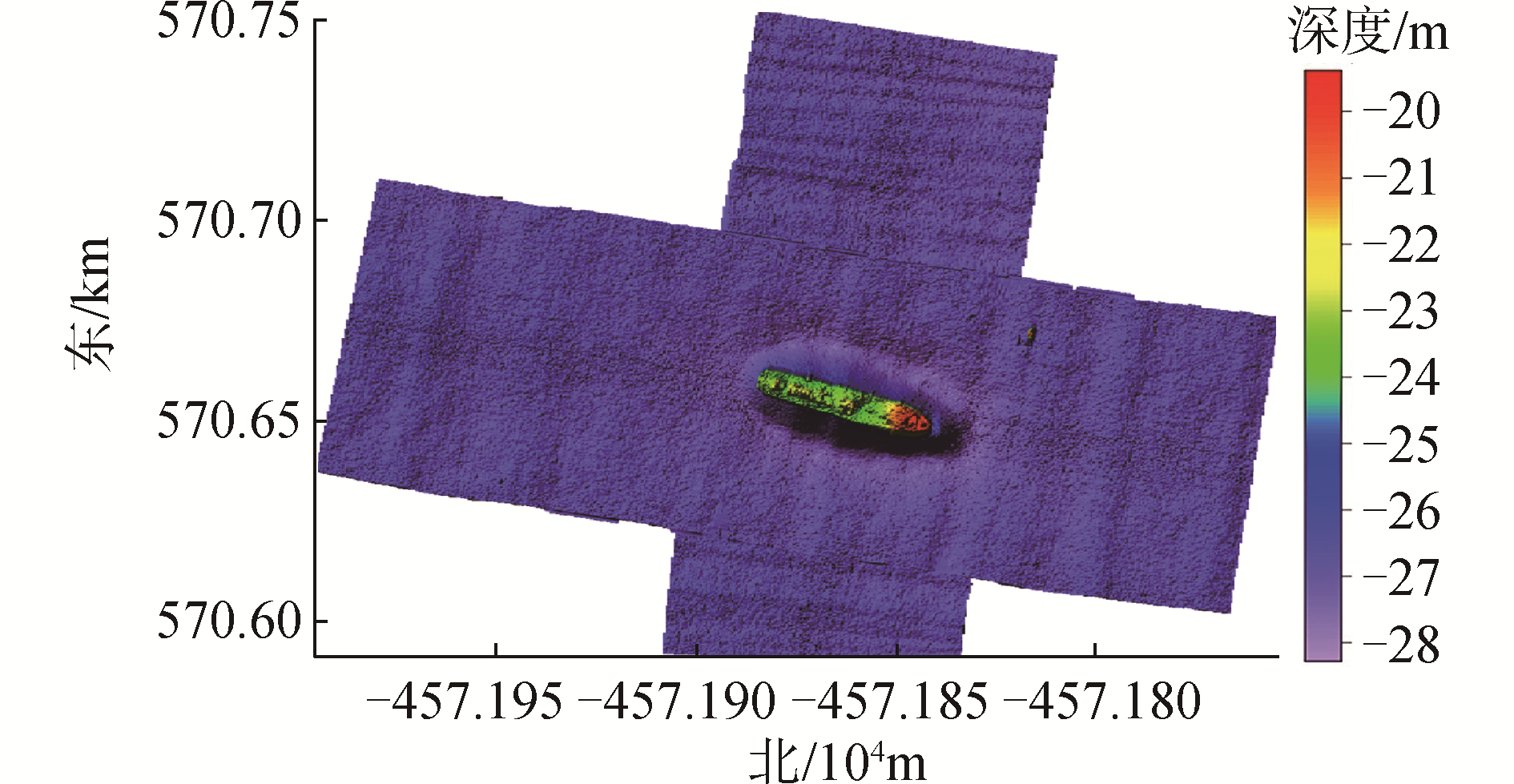Download: 图 4 MB-system处理后得到的浅水区光照地形图 Fig. 4 Sun illuminate, depth colored grid of the shallow water data processed by MB-system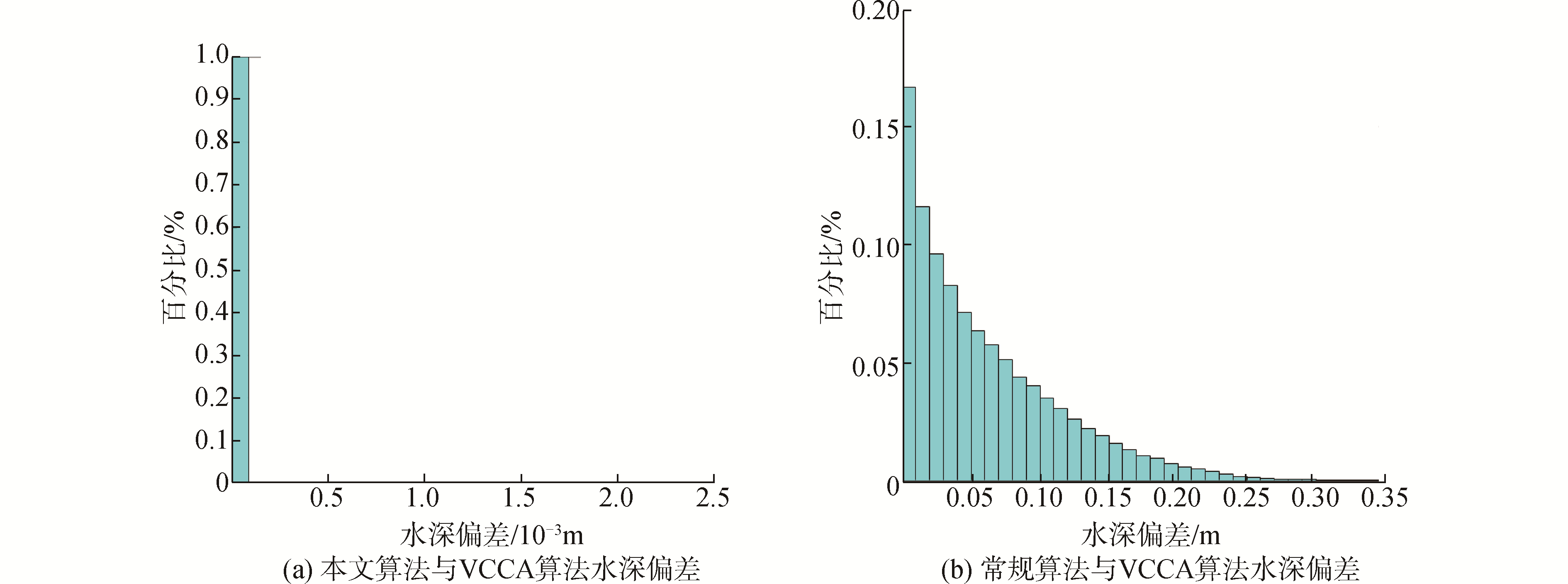Download: 图 5 浅水数据水深偏差统计 Fig. 5 Statistics of the depth deviations with shallow water data表 1 浅水数据测深点误差统计 Table 1 Error statistics of the soundings form the shallow water data表 2 深度不符值统计分析 Table 2 Statistical analysis of depth discrepancy

3.2 深水数据实验分析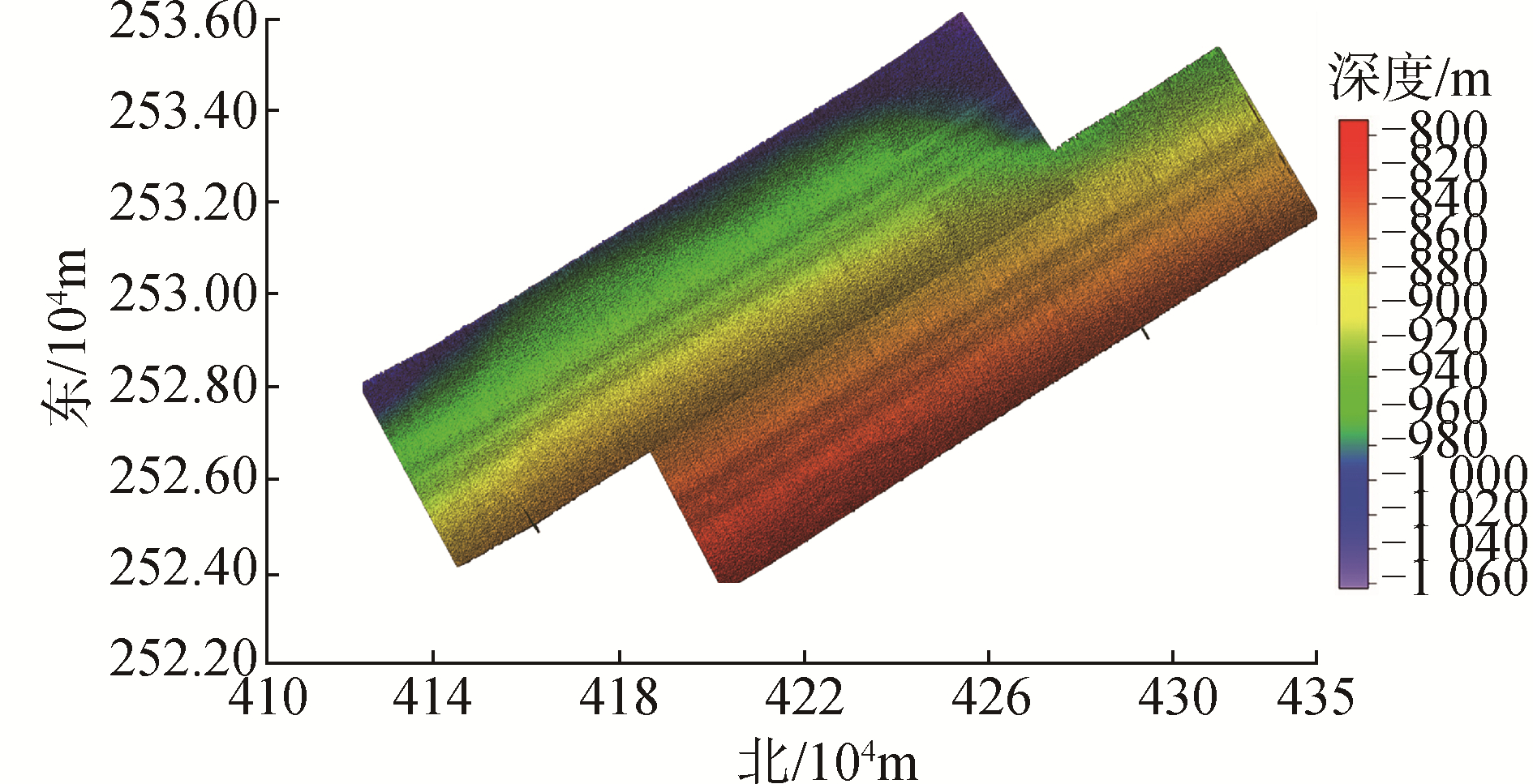Download: 图 6 MB-system处理后得到的深水区光照地形图 Fig. 6 Sun illuminate, depth colored grid of the deep water data processed by MB-system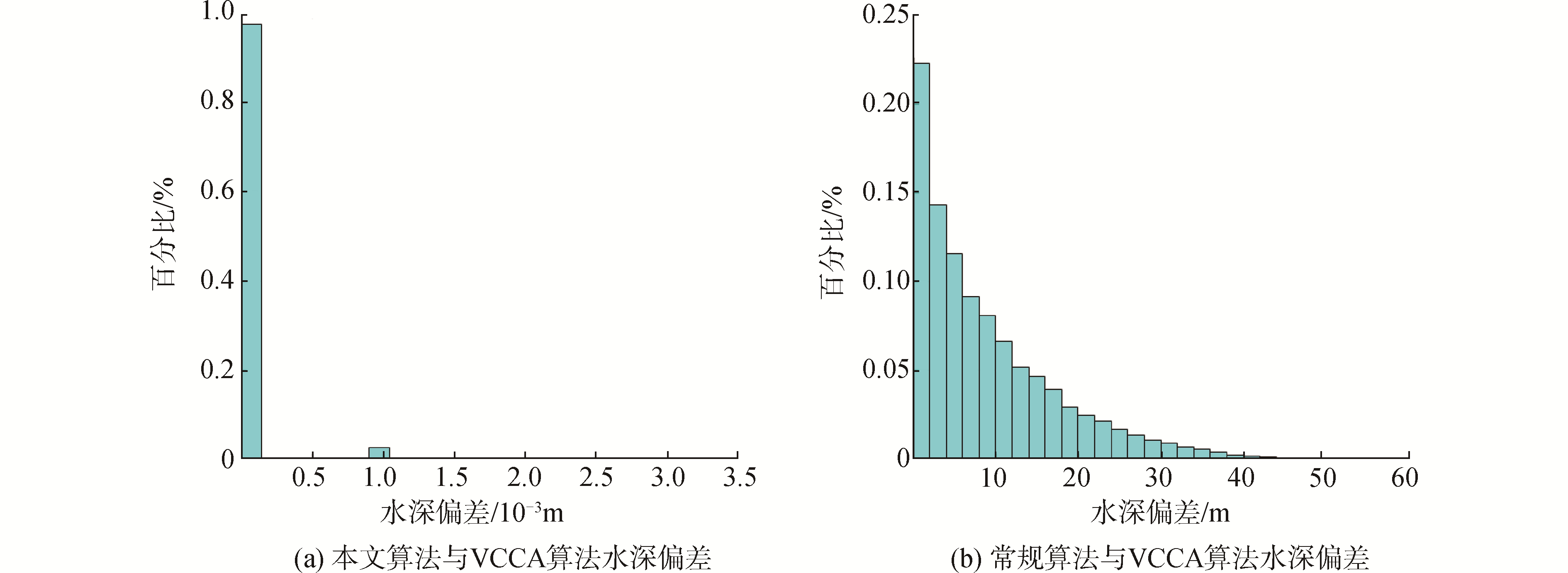Download: 图 7 深水数据水深偏差统计 Fig. 7 Statistics of the depth deviations with deep water data表 3 深水数据测深点误差统计 Table 3 Error statistics of the soundings form the deep water data表 4 深度不符值统计分析 Table 4 Statistical analysis of depth discrepancy

4 结论

1) 多波束测深波束往返期间发射换能器的姿态变化产生的影响在浅水区相对较小，因此，常规模型只适用于浅水区；当测区水深增加时，该影响产生的误差逐渐增大，使用常规模型易导致测深点位置整体出现偏差且水深误差易超出1%的测深精度要求。

2) 本文基于VCCA算法，提出一种同心双曲线相交的波束归位算法，将波束脚印作为发射圆锥面和接收圆锥面与交点平面投影形成的两双曲线交点，并推导了双曲线参数定义与解算方法，从而将VCCA算法中复杂的波束向量推导过程转化为求解相交双曲线方程的形式，模型更加直观且易于理解。

3) 使用实测数据对本文算法进行验证，结果表明，常规波束归位算法由于忽略波束往返期间换能器的姿态变化，使得数据处理结果存在误差，且随着水深的逐渐增加误差也逐渐增大，因此常规算法仅适用于处理浅水多波束测量数据；本文算法对浅水与深水多波束数据的计算结果与VCCA算法数据处理结果基本一致，计算结果满足1%的水深测量精度要求，因此本文算法具有有效性，对后续多波束数据处理研究具有很好的参考性。

  赵建虎, 刘经南. 精密多波束测深系统位置修正方法研究[J]. 武汉大学学报(信息科学版), 2002, 27(5): 473-477. ZHAO Jianhu, LIU Jingnan. Development of method in precise multibeam acoustic bathymetry[J]. Geomatics and Information Science of Wuhan University, 2002, 27(5): 473-477. (0)  李家彪. 多波束勘测原理技术与方法[M]. 北京: 海洋出版社, 1999. (0)  赵建虎, 沈文周, 吴永亭, 等. 现代海洋测绘[M]. 武汉: 武汉大学出版社, 2011. (0)  阳凡林, 暴景阳, 胡兴树, 等. 水下地形测量[M]. 武汉: 武汉大学出版社, 2017. (0)  赵建虎, 刘经南. 多波束测深系统的归位问题研究[J]. 海洋测绘, 2003, 23(1): 6-7, 12. ZHAO Jianhu, LIU Jingnan. Problems on the conformity to the real sounding points from the multi-beam sounding system[J]. Hydrographic surveying and charting, 2003, 23(1): 6-7, 12. DOI:10.3969/j.issn.1671-3044.2003.01.002 (0)  黄谟涛, 翟国君, 谢锡君, 等. 多波束和机载激光测深位置归算及载体姿态影响研究[J]. 测绘学报, 2000, 29(1): 82-88. HUANG Motao, ZHAI Guojun, XIE Xijun, et al. The influence of carrier's attitude and the position reduction in multibeam echosounding and airborne laser depth sounding[J]. Acta geodaetica et cartographica sinica, 2000, 29(1): 82-88. DOI:10.3321/j.issn:1001-1595.2000.01.014 (0)  阳凡林, 李家彪, 吴自银, 等. 多波束测深瞬时姿态误差的改正方法[J]. 测绘学报, 2009, 38(5): 450-456. YANG Fanlin, LI Jiabiao, WU Ziyin, et al. The methods of removing instantaneous attitude errors for multibeam bathymetry data[J]. Acta geodaetica et cartographica sinica, 2009, 38(5): 450-456. DOI:10.3321/j.issn:1001-1595.2009.05.012 (0)  何林帮, 赵建虎, 张红梅, 等. 顾及姿态角的多波束声线精确跟踪方法[J]. 哈尔滨工程大学学报, 2015, 36(1): 46-50. HE Linbang, ZHAO Jianhu, ZHANG Hongmei, et al. A precise multibeam sound ray tracking method taking into account the attitude angle[J]. Journal of Harbin Engineering University, 2015, 36(1): 46-50. (0)  杨绍海, 张彦昌. 多波束测量中海底测点三维坐标计算方法研究[J]. 气象水文海洋仪器, 2011, 28(2): 1-3, 19. YANG Shaohai, ZHANG Yanchang. Study on coordinate calculation of seabed point on multi-beam surveying[J]. Meteorological, hydrological and marine instruments, 2011, 28(2): 1-3, 19. DOI:10.3969/j.issn.1006-009X.2011.02.001 (0)  朱小辰, 刘雁春, 肖付民, 等. 多波束测深波束脚印位置归算模型研究[J]. 海洋测绘, 2011, 31(5): 24-27. ZHU Xiaochen, LIU Yanchun, XIAO Fumin, et al. The research of footprint location determination model of multibeam echosoundings[J]. Hydrographic surveying and charting, 2011, 31(5): 24-27. DOI:10.3969/j.issn.1671-3044.2011.05.008 (0)  阳凡林, 卢秀山, 李家彪, 等. 多波束勘测运动传感器偏移的改正方法[J]. 武汉大学学报(信息科学版), 2010, 35(7): 816-820. YAN Fanlin, LU Xiushan, LI Jiabiao, et al. Correction of imperfect alignment of MRU for multibeam bathymetry data[J]. Geomatics and Information Science of Wuhan University, 2010, 35(7): 816-820. (0)  BEAUDOIN J D, HUGHES CLARKE J E, BARTLETT J E. Application of surface sound speed measurements in post-processing for multi-sector multibeam echosounders[J]. The international hydrographic review, 2004, 5(3): 17-32. (0)  HAMILTON T, BEAUDOIN J, CLARKE J H. A more precise algorithm to account for non concentric multibeam array geometry[C]//Proceedings of Canadian Hydrographic Conference.[S.l.], 2014. (0)  TENG Y. Sector-specific beam pattern compensation for multi-sector and multi-swath multibeam sonars[D]. New Brunswick: University of New Brunswick, 2011. (0)  罗才忠. 关于Dandelin定理的证明[J]. 数学通报, 2004(10): 6. LUO Caizhong. Proof of Dandelin's theorem[J]. Bulletin des sciences mathematics, 2004(10): 6. DOI:10.3969/j.issn.0583-1458.2004.10.004 (0)  郭启淳. 圆锥曲线的学习总结[J]. 中国校外教育, 2018(3): 120-121. GUO Qichun. Learning summary of conic curves[J]. Education for Chinese after-school, 2018(3): 120-121. DOI:10.3969/j.issn.1004-8504.2018.03.094 (0)  卜宪海, 王明伟, 阳凡林, 等. 多波束测深波束归位近似转换模型研究[J]. 山东科技大学学报(自然科学版), 2016, 35(5): 28-34. BU Xianhai, WANG Mingwei, YANG Fanlin, et al. Research on approximate conversion model of beam homing in multibeam echosounding[J]. Journal of Shandong University of Science and Technology (natural science), 2016, 35(5): 28-34. DOI:10.3969/j.issn.1672-3767.2016.05.004 (0)  曲萌, 樊妙, 卜宪海, 等. 基于严密波束归位模型的多波束测深点不确定度改进方法[J]. 海洋通报, 2018, 37(6): 643-651. QU Meng, FAN Miao, BU Xianhai, et al. Uncertainty calculation of multibeam echo sounder based on accurate reduction model of footprints[J]. Marine science bulletin, 2018, 37(6): 643-651. (0)  卜宪海.基于激光点云约束的多波束低掠射波束几何改正[D].青岛: 山东科技大学, 2017. BU Xianhai. Geometric calibration method for the multibeam bathymetric data at large incidence angles based on the laser tie points[D]. Qingdao: Shandong University of Science and Technology, 2017. (0)  中华人民共和国交通运输部. JTS 131-2012, 水运工程测量规范[S].北京: 人民交通出版社, 2013. Ministry of Transport of the People's Republic of China. JTS 131-2012, Specifications for port and waterway engineering survey[S]. Beijing: People's Communications Press, 2013. (0)